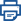Print this pageTitle

# Kyoritsu Course – The Charm of Mathematics 11Gendai Suri Tokei-gaku no Kiso(Foundations of Modern Mathematical Statistics)

Size

324 pages, A5 format

Language

Japanese

Released

April, 2017

ISBN

978-4-320-11166-0

Kyoritsu Shuppan

Book Info

See Book Availability at Library

Japanese Page

view japanese page

Extracting useful information from data for use in decision-making and other future behavior is important in a variety of fields. In recent years, terms such as Big Data and data science have been heard increasingly often and the value of data and the importance of data analysis have become widely recognized.

Data can be viewed as a series of numbers, but the real pleasure of statistics is in going beyond mere series of numbers. What is therefore important is a concept known as a probability distribution. In the field of statistical inference, data is regarded as a probabilistic phenomenon that is accompanied by randomness. To state this another way, data is produced as the result of trends. Statistical inference is a way of thinking that involves modeling the probability distribution of a trend and perceiving that data occurs according to that probability distribution. Statistical mathematics is the field of study that provides the mathematical basis for this way of thinking. Accordingly, it becomes possible to depict the intellectual world of the probability distribution or probabilistic model that exists behind the data, and to extract the truths that are hidden there. The pleasure of statistics is that data can be transformed in this way, from a mere series of numbers to an intellectual asset.

Many excellent textbooks on statistical mathematics have already been published, but what distinguishes this book is the inclusion of computational statistical concepts such as the Markov chain Monte Carlo methods, bootstrapping, EM algorithms, as well as concepts that have been widely used in recent years, such as variable selection methods for linear regression models, logistic regression models, and so on.

This book is composed of three parts. The first part addresses the basics of probability and probability distributions; the second part deals with statistical methods for inference in probability distributions, such as sample distribution, statistical estimation, and hypothesis testing. The material covered up to this point forms the foundation of mathematical statistics, the material studied in the third and fourth years of an economics major. The third part deals with content that is currently evolving, such as linear regression models, risk optimization theory, computational statistical methods, and stochastic processes, material that should be learned from the fourth year of undergraduate studies to the first year of a master’s course. Furthermore, as a useful tool in deepening the reader’s understanding of the concepts, a wealth of end-of-chapter problems have been prepared with answers available at: https://sites.google.com/site/ktatsuya77/.

This book has been written to focus on mathematically dealing with the contents of inferential statistics, an area of study within statistics itself. Mathematical understanding, along with helping us to understand statistical methods more deeply, can cultivate the skills necessary to respond flexibly to the development and application of new statistical methods in the future. However, to truly learn “living statistics,” it is important to study the material while keeping specific images of data analysis in mind. As a result, We recommend reading this book after having used an introductory book on statistics that is filled with concrete application examples to learn the true pleasures, usefulness, and motivations behind data analysis.

(Written by KUBOKAWA Tatsuya, Professor, Graduate School of Economics / 2018)